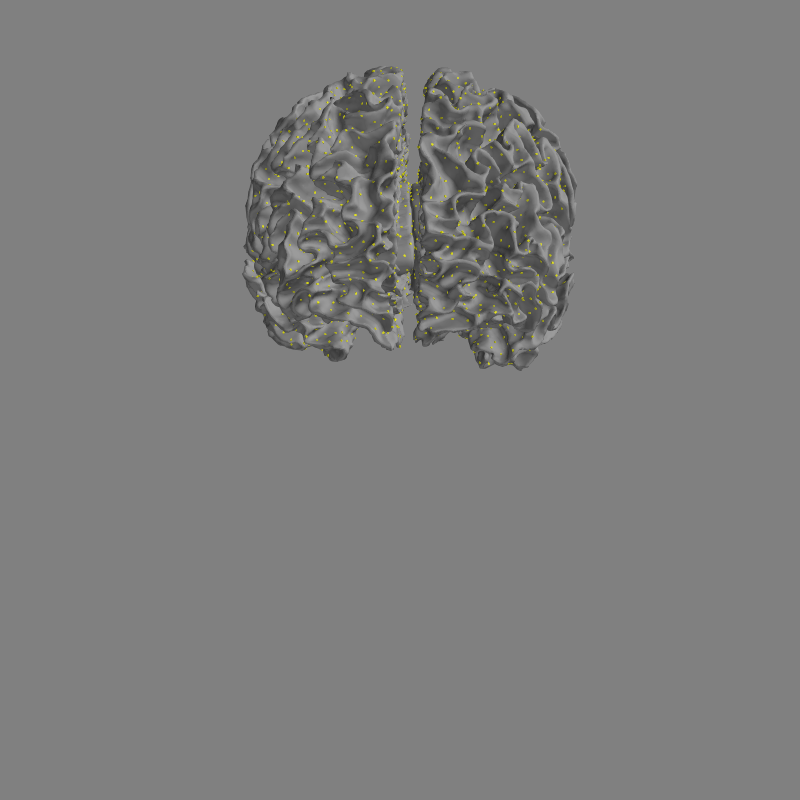The inverse operator’s source space is shown in 3D.

# Author: Alexandre Gramfort <alexandre.gramfort@inria.fr>
#

import mne
from mne.datasets import sample
from mne.viz import set_3d_view

print(__doc__)

data_path = sample.data_path()
subjects_dir = data_path + '/subjects'

fname_trans = data_path + '/MEG/sample/sample_audvis_raw-trans.fif'

inv_fname = data_path
inv_fname += '/MEG/sample/sample_audvis-meg-oct-6-meg-inv.fif'

print("Method: %s" % inv['methods'])
print("fMRI prior: %s" % inv['fmri_prior'])
print("Number of sources: %s" % inv['nsource'])
print("Number of channels: %s" % inv['nchan'])

src = inv['src']  # get the source space

print("Number of vertices on the left hemisphere: %d" % len(src['rr']))
print("Number of triangles on left hemisphere: %d" % len(src['use_tris']))
print("Number of vertices on the right hemisphere: %d" % len(src['rr']))
print("Number of triangles on right hemisphere: %d" % len(src['use_tris']))


Out:

Reading inverse operator decomposition from /home/circleci/mne_data/MNE-sample-data/MEG/sample/sample_audvis-meg-oct-6-meg-inv.fif...
[done]
[done]
305 x 305 full covariance (kind = 1) found.
Read a total of 4 projection items:
PCA-v1 (1 x 102) active
PCA-v2 (1 x 102) active
PCA-v3 (1 x 102) active
Average EEG reference (1 x 60) active
22494 x 22494 diagonal covariance (kind = 2) found.
22494 x 22494 diagonal covariance (kind = 6) found.
22494 x 22494 diagonal covariance (kind = 5) found.
Did not find the desired covariance matrix (kind = 3)
Computing patch statistics...
[done]
Computing patch statistics...
[done]
Read a total of 4 projection items:
PCA-v1 (1 x 102) active
PCA-v2 (1 x 102) active
PCA-v3 (1 x 102) active
Average EEG reference (1 x 60) active
Source spaces transformed to the inverse solution coordinate frame
Method: 1
fMRI prior: None
Number of sources: 7498
Number of channels: 305
Number of vertices on the left hemisphere: 155407
Number of triangles on left hemisphere: 8192
Number of vertices on the right hemisphere: 156866
Number of triangles on right hemisphere: 8192


Show result on 3D source space

fig = mne.viz.plot_alignment(subject='sample', subjects_dir=subjects_dir,
trans=fname_trans, surfaces='white', src=src)
set_3d_view(fig, focalpoint=(0., 0., 0.06))Total running time of the script: ( 0 minutes 2.719 seconds)

Estimated memory usage: 13 MB

Gallery generated by Sphinx-Gallery